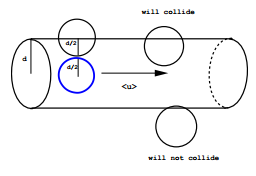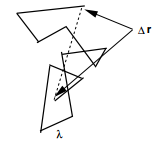13.2: Collision Theory

Collision energy

Consider two particles $$A$$ and $$B$$ in a system. The kinetic energy of these two particles is

$K_{AB} = \dfrac{\textbf{p}_A^2}{2m_A} + \dfrac{\textbf{p}_B^2}{2m_B} \label{6}$

Let us change to center-of-mass $$\left( \textbf{P} \right)$$ and relative $$\left( \textbf{p} \right)$$ momenta, which are given by

$\textbf{P} = \textbf{p}_A + \textbf{p}_B, \: \: \: \textbf{p} = \dfrac{m_B \textbf{p}_a - m_A \textbf{p}_B}{M} \label{7}$

where $$M = m_A + m_B$$ is the total mass of the two particles. Substituting this into the kinetic energy, we find

$K_{AB} = \dfrac{\textbf{p}_A^2}{2m_A} + \dfrac{\textbf{p}_B^2}{2m_B} = \dfrac{\textbf{P}^2}{2M} + \dfrac{\textbf{p}^2}{2 \mu} \label{8}$

where

$\mu = \dfrac{m_A m_B}{M} \label{9}$

is called the reduced mass of the two particles. Note that the kinetic energy separates into a sum of a center-of-mass term and a relative term.

Now the relative position is $$\textbf{r} = \textbf{r}_A - \textbf{r}_B$$ so that the relative velocity is $$\dot{\textbf{r}} = \dot{\textbf{r}}_A - \dot{\textbf{r}}_B$$ or $$\textbf{v} = \textbf{v}_A - \textbf{v}_B$$. Thus, if the two particles are approaching each other such that $$\textbf{v}_A = - \textbf{v}_B$$, then $$\textbf{v} = 2 \textbf{v}_A$$. However, by equipartitioning the relative kinetic energy, being mass independent, is

$\left< \dfrac{\textbf{p}^2}{2 \mu} \right> = \dfrac{3}{2} k_B T \label{10}$

which is called the collision energy

Collision cross section

Consider two molecules in a system. The probability that they will collide increases with the effective “size” of each particle. However, the size measure that is relevant is the apparent cross-section area of each particle. For simplicity, suppose the particles are spherical, which is not a bad approximation for small molecules. If we are looking at a sphere, what we perceive as the size of the sphere is the cross section area of a great circle. Recall that each spherical particle has an associated “collision sphere” that just encloses two particles at closest contact, i.e., at the moment of a collision, and that this sphere is a radius $$d$$, where $$d$$ is the diameter of each spherical particle (see lecture 5). The cross-section of this collision sphere represents an effective cross section for each particle inside which a collision is imminent. The cross-section of the collision sphere is the area of a great circle, which is $$\pi d^2$$. We denote this apparent cross section area $$\sigma$$. Thus, for spherical particles $$A$$ and $$B$$ with diameters $$d_A$$ and $$d_B$$, the individual cross sections are

$\sigma_A = \pi d_A^2, \: \: \: \sigma_B = \pi d_B^2 \label{11}$

The collision cross section, $$\sigma_{AB}$$ is determined by an effective diameter $$d_{AB}$$ characteristic of both particles. The collision probability increases of both particles have large diameters and decreases if one of them has a smaller diameter than the other. Hence, a simple measure sensitive to this is the arithmetic average

$d_{AB} = \dfrac{1}{2} \left( d_A + d_B \right) \label{12}$

and the resulting collision cross section becomes

\begin{align} \sigma_{AB} &= \pi d_{AB}^2 \\ &= \pi \left( \dfrac{d_A + d_B}{2} \right)^2 \\ &= \dfrac{\pi}{4} \left( d_A^2 + 2d_A d_B + d_B^2 \right) \\ &= \dfrac{1}{4} \left( \sigma_A + 2 \sqrt{\sigma_A \sigma_B} + \sigma_B \right) \\ &= \dfrac{1}{2} \left[ \left( \dfrac{\sigma_A + \sigma_B}{2} \right) + \sqrt{\sigma_A \sigma_B} \right] \end{align} \label{13}

which, interestingly, is an average of the two types of averages of the two individual cross sections, the arithmetic and geometric averages!

Average collision Frequency

Consider a system of particles with individual cross sections $$\sigma$$. A particle of cross section $$\sigma$$ that moves a distance $$l$$ in a time $$\Delta t$$ will sweep out a cylindrical volume (ignoring the spherical caps) of volume $$\sigma l$$ (Figure $$\PageIndex{1}$$). If the system has a number density $$\rho$$, then the number of collisions that will occur is

$N_{\text{coll}} = \rho \sigma l \label{14}$Figure $$\PageIndex{1}$$: Collision cylinder. Any particle that partially overlaps with this volume will experience a collision with a test particle tracing out this volume.

We define the average collision rate as $$N_{\text{coll}}/ \Delta t$$, i.e.,

$\gamma = \dfrac{N_{\text{coll}}}{\Delta t} = \dfrac{\rho \sigma l}{\Delta t} = \rho \sigma \langle | \textbf{v} | \rangle \label{15}$

where $$\langle | \textbf{v} | \rangle$$ is the average relative speed. If all of the particles are of the same type (say, type $$A$$), then performing the average over a Maxwell-Boltzmann speed distribution gives

$\langle | \textbf{v} | \rangle = \sqrt{\dfrac{8 k_B T}{\pi \mu}} \label{16}$

where $$\mu = m_A/2$$ is the reduced mass. The average speed of a particle is

$\langle | \textbf{v}_A | \rangle = \sqrt{\dfrac{8 k_B T}{\pi m_A}} \label{17}$

so that

$\langle | \textbf{v} | \rangle = \sqrt{2} \langle | \textbf{v}_A | \rangle \label{18}$

Mean Free Path

The mean free path is the distance a particle will travel, on average, before experiencing a collision event. This is defined as the product of the speed of a particle and the time between collisions. The former is $$\langle | \textbf{v} | \rangle/ \sqrt{2}$$, while the latter is $$1/\gamma$$. Hence, we have

$\lambda = \dfrac{\langle | \textbf{v} |\rangle}{\sqrt{2} \rho \sigma \langle | \textbf{v} | \rangle} = \dfrac{1}{\sqrt{2} \rho \sigma} \label{19}$

Random Walks

In any system, a particle undergoing frequent collisions will have the direction of its motion changed with each collision and will trace out a path that appears to be random. In fact, if we treat the process as statistical, then, we are, in fact, treating each collision event as a random event, and the particle will change its direction at random times in random ways! Such a path might appear as shown in Figure $$\PageIndex{2. Such a path is often referred to as a random walk path.Figure \(\PageIndex{2}$$: Random walk path.

In order to analyze such paths, let us consider a random walk in one dimension. We’ll assume that the particle move a mean-free path length $$\lambda$$ between collisions and that each collision changes the direction of the particles motion, which in one dimension, means that the particle moves either to the right or to the left after each event. This can be mapped onto a metaphoric “coin toss” that can come up heads “H” or tails “T”, with “H” causing motion to the right, and “T” causing motion to the left. Let there be $$N$$ such coin tosses, let $$i$$ be the number of times “H” comes up and $$j$$ denote the number of times “T” comes up. Thus, the progress of the particle, which we define as net motion to the right, is given by $$(i - j) \lambda$$. Letting $$k = i - j$$, this is just $$k \lambda$$. Thus, we need to know what the probability is for obtaining a particular value of $$k$$ in a very large number $$N$$ of coin tosses. Denote this $$P(k)$$.

In $$N$$ coin tosses, the total number of possible sequences of “H” and “T” is $$2^N$$. However, the number of ways we can obtain $$i$$ heads and \)j\) tails, with $$i + j = N$$ is a binomial coefficient $$N!/i!j!$$. Now

$j = N - i = N - (j + k) = N - j - k \label{20}$

so that $$j = (N - k )/2$$. Similarly,

$i = N - j = N - (i - k) = N - i + k \label{21}$

so that $$i = (N + k)/2$$. Thus, the probability $$P(k)$$ is

$P(k) = \dfrac{N!}{2^N i! j!} = \dfrac{1}{2^N} \dfrac{N!}{\left(\dfrac{N + k}{2} \right) ! \left( \dfrac{N - k}{2} \right) !} \label{22}$

We now take the logarithm of both sides:

$\text{ln} \: P(k) = \: \text{ln} \: N! - \: \text{ln} \: 2^N - \: \text{ln} \: \left( \dfrac{N + k}{2} \right) ! - \: \text{ln} \: \left( \dfrac{N - k}{2} \right) ! \label{23}$

and use Stirling’s approximation:

$\text{ln} \: N! \approx N \: \text{ln} \: N - N \label{24}$

and write $$\text{ln} \: P(k)$$ as

\begin{align} \text{ln} \: P(k) &\approx N \: \text{ln} \: N - N - N \: \text{ln} \: 2 - \dfrac{1}{2} (N + k) \: \text{ln} \: \dfrac{1}{2} (N + k) + \dfrac{1}{2} (N + k) - \dfrac{1}{2} (N - k) \: \text{ln} \: \dfrac{1}{2} (N - k) + \dfrac{1}{2} (N - k) \\ &= N \: \text{ln} \: N - N \: \text{ln} \: 2 + \dfrac{1}{2} (N + k) \: \text{ln} \: \dfrac{1}{2} - \dfrac{1}{2} (N + k) \: \text{ln} \: (N + k) - \dfrac{1}{2} (N - k) \: \text{ln} \: \dfrac{1}{2} - \dfrac{1}{2} (N - k) \: \text{ln} \: (N - k) \\ &= N \: \text{ln} \: N - N \: \text{ln} \: 2 + \dfrac{1}{2} (N + k) \: \text{ln} \: 2 - \dfrac{1}{2} (N + k) \: \text{ln} \: (N + k) + \dfrac{1}{2} (N - k) \: \text{ln} \: 2 - \dfrac{1}{2} (N - k) \: \text{ln} \: (N - k) \\ &= N \: \text{ln} \: N - \dfrac{1}{2} \left[ (N + k) \: \text{ln} \: (N + k) + (N - k) \: \text{ln} \: (N - k) \right] \end{align} \label{25}

Now, write

$\text{ln} \: (N + k) = \: \text{ln} \: N \left( 1 + \dfrac{k}{N} \right) = \: \text{ln} \: N + \: \text{ln} \: \left( 1 + \dfrac{k}{N} \right) \label{26}$

and

$\text{ln} \: (N - k) = \: \text{ln} \: N \left( 1 - \dfrac{k}{N} \right) = \: \text{ln} \: N + \: \text{ln} \: \left( 1 - \dfrac{k}{N} \right) \label{27}$

We now use the expansions

\begin{align} \text{ln} \: \left( 1 + \dfrac{k}{N} \right) &= \left( \dfrac{k}{N} \right) - \dfrac{1}{2} \left( \dfrac{k}{N} \right)^2 + \cdots \\ \text{ln} \: \left( 1 - \dfrac{k}{N} \right) &= - \left( \dfrac{k}{N} \right) - \dfrac{1}{2} \left( \dfrac{k}{N} \right)^2 + \cdots \end{align} \label{28}

If we stop at the second-order term, then

\begin{align} \text{ln} \: P(k) &= N \: \text{ln} \: N - \dfrac{1}{2} (N + k) \left[ \: \text{ln} \: N + \left( \dfrac{k}{N} \right) - \dfrac{1}{2} \left( \dfrac{k}{N} \right)^2 \right] - \dfrac{1}{2} (N - k) \left[ \: \text{ln} \: N - \left( \dfrac{k}{N} \right) - \dfrac{1}{2} \left( \dfrac{k}{N} \right)^2 \right] \\ &= -\dfrac{1}{2} (N + k) \left[ \left( \dfrac{k}{N} \right) - \dfrac{1}{2} \left( \dfrac{k}{N} \right)^2 \right] + \dfrac{1}{2} (N - k) \left[ \left( \dfrac{k}{N} \right) + \dfrac{1}{2} \left( \dfrac{k}{N} \right)^2 \right] \\ &= \dfrac{1}{2} N \left( \dfrac{k}{N} \right)^2 - k \left( \dfrac{k}{N} \right) \\ & = \dfrac{k^2}{2N} - \dfrac{k^2}{N} = - \dfrac{k^2}{2N} \end{align} \label{29}

so that

$P(k) = e^{-k^2/2N} \label{30}$

Now, if we let $$x = k \lambda$$ and $$L = \sqrt{N} \lambda$$, and if we let $$x$$ be a continuous random variable, then the corresponding probability distribution $$P(x)$$ becomes

$P(x) = \dfrac{1}{L \sqrt{2 \pi}} \: e^{-x^2/2L^2} = \dfrac{1}{\sqrt{2 \pi N \lambda^2}} \: e^{-x^2/2N \lambda^2} \label{31}$

which is a simple Gaussian distribution. Now, $$N$$ is the number of collisions, which is given by $$\gamma t$$, so we can write the probability distribution for the particle to diffuse a distance $$x$$ in time $$t$$ as

$P(x, t) = \dfrac{1}{\sqrt{2 \pi \gamma t \lambda^2}} \: e^{-x^2/2 \gamma t \lambda^2} \label{32}$

Define $$D = \gamma \lambda^2/2$$ as the diffusion constant, which has units of (length)$$^{\text{2}}$$/time. The distribution then becomes

$P(x, t) = \dfrac{1}{\sqrt{4 \pi D t }} \: e^{-x^2/4D t} \label{33}$

Note that this distribution satisfies the following equation:

$\dfrac{\partial}{\partial t} P(x, t) = D \dfrac{\partial^2}{\partial x^2} P(x, t) \label{34}$

which is called the diffusion equation. The diffusion equation is, in fact, more general than the Gaussian distribution in Equation $$\ref{33}$$. It is capable of predicting the distribution in any one-dimensional geometry subject to any initial distribution $$P(x, 0)$$ and any imposed boundary conditions.

In three dimensions, we consider the three spatial directions to be independent, hence, the probability distribution for a particle to diffuse to a location $$\textbf{r} = (x, y, z)$$ is just a product of the three one-dimensional distributions:

$\mathcal{P}(\textbf{r}) = P(x) \: P(y) \: P(z) = \dfrac{1}{(4 \pi D t) ^{3/2}} \: e^{-\left( x^2 + y^2 + z^2 \right)/4Dt} \label{35}$

and if we are only interested in diffusion over a distance $$r$$, we can introduce spherical coordinates, integrate over the angles, and we find that

$P(r, t) = \dfrac{4 \pi}{(4 \pi D t)^{3/2}} \: E^{-r^2/4Dt} \label{36}$